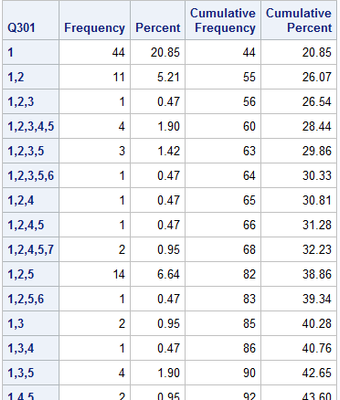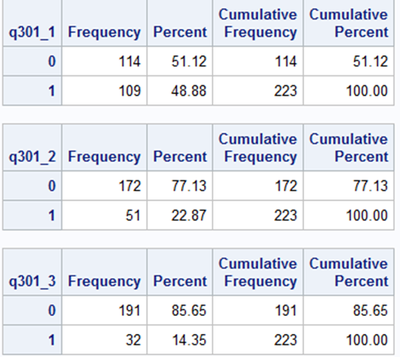## Creating binary variables that combine answer choices from "Select all that apply"

Hello all,

I am having trouble finding a way to create binary variables from a “select all that apply” survey question in order to run logistic regression.

The question asks about factors that inhibit health care use, with the option to choose more than one answer choice out of 8 possible choices (e.g., “Concerned about quality of care,” “Concerned about privacy,” etc.).

I would like to combine certain answer choices and create binary variables to represent them. For example, the first binary variable would represent concerns about quality of care from answer choices (1) and (2), all other responses that do not contain (1), (2), or both, would be coded as 0 (i.e., no concerns about quality of care).

The second binary variable would represent concerns about privacy from answer choices (3) and (4), all other answer choices that do not contain (3), (4), or both, would be coded as 0 (i.e., no concerns about privacy).

My goal is complicated by the fact that some respondents selected choices (1), (2), (3), and/or (4) at once. As an example, here is a snippet of what the raw frequencies look like:I was able to separate each answer choice into its own variable using the following code:

``````data &health;
set &health;
array q301_ ;
do index=1 to 8;
q301_[index]=0 ne findw(q301,cats(index),',','t');
end;
drop index;
run;``````

Some of the output was as follows:However, given that this is “select all that apply”, I’m not sure how to manipulate the data to create binary variables that combine answer choices as described above. Is this possible?

1 ACCEPTED SOLUTION

Accepted Solutions

## Re: Creating binary variables that combine answer choices from "Select all that apply"

After you have the individual response binaries then you create additional variables by using the information in them.

You are not very careful in describing exactly how you want to use the responses but if you want to know if two or more individual response variables have been selected consider:

r_34 = (sum (q301_3,q301_4) = 2 );

If all of the variables have a value of 1 then the sum will be the number of variables.

A similar check for a sum of 0 for "none" were selected may be of use.

You check to see if ANY of the list were selected by Max(<variable list>) = 1.

None selected would be Sum(<variable list>)=0.

All the same, value not needed:  Range(<variable list>)=0

5 REPLIES 5AhmedAl_Attar
Rhodochrosite | Level 12

## Re: Creating binary variables that combine answer choices from "Select all that apply"

Have you thought about creating and using custom formats?

SAS® Fundamentals For Survey Data Processing

You could create separate format per question, where you can change the transformation of the answers based on your custom scaling and the question at hand.

Hope this helps

## Re: Creating binary variables that combine answer choices from "Select all that apply"

I don't understand.  You asked how to create binary variables.  Then you showed how to create the binary variables.

What is it that you want that is different than what you already showed how to do?

## Re: Creating binary variables that combine answer choices from "Select all that apply"

Apologies for the confusion. I posted that code in case there was some way to modify it to get what I was looking for. The suggestions from @ballardw helped.

## Re: Creating binary variables that combine answer choices from "Select all that apply"

After you have the individual response binaries then you create additional variables by using the information in them.

You are not very careful in describing exactly how you want to use the responses but if you want to know if two or more individual response variables have been selected consider:

r_34 = (sum (q301_3,q301_4) = 2 );

If all of the variables have a value of 1 then the sum will be the number of variables.

A similar check for a sum of 0 for "none" were selected may be of use.

You check to see if ANY of the list were selected by Max(<variable list>) = 1.

None selected would be Sum(<variable list>)=0.

All the same, value not needed:  Range(<variable list>)=0

## Re: Creating binary variables that combine answer choices from "Select all that apply"

This is did the trick perfectly, thank you so much! I have about 30+ "select all that apply" questions to work with, so this will be incredibly helpful. Much appreciated.

Discussion stats
• 5 replies
• 393 views
• 4 likes
• 4 in conversation